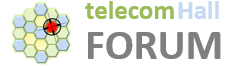# Introduction

The development of modulation techniques allowed significant developments in mobile communications through the transmission of symbols carrying a large amount of information.

However, there is a common problem in such systems: the interference inter symbols (ISI or Inter Symbol Interference).Let us understand this today: what is the interference between symbols (ISI), existing in the LTE Systems, and also in any technology that uses symbols for the information transport.

# What is ISI?

In an ideal system (theoretical), the transmitted symbols arrive at the receiver without any loss or interference, as shown in the following figure.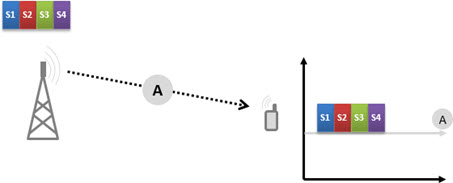But in a real scenario the transmitted signals are affected in different ways, for example, according to the propagation environment.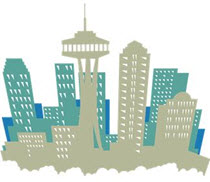What happens in practice is that the “same” signal arrives via multiple paths (“Multipath”) and consequently with different delays (“Delay Spread”).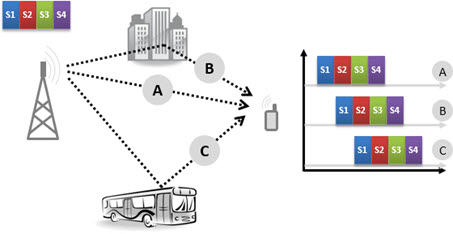Although the “Multipath” bring positive benefits, “Multipath” and “Delay Spread” also end up causing interference inter symbols.

So let’s learn about these factors.

A transmitted symbol can be received multiple times at the receiver, more or less as an “echo” effect. This echo is what we call “Delay Spread”.In the above figure, the transmitter transmits a single symbol. This symbol is propagated along different paths (A, B and C), and eventually reaching the receiver at multiple time instants, and therefore with multiple “replication.”

The total elapsed time between the first and last is determined by the environment (including the structures, how close they are, etc…). For example, in an urban environment, where the reflection is high (many buildings, many vehicles parked and moving), this delay has a typical value of 5-10 microseconds.

# Multipath

And as we talked about before, in a theoretical ideal scenario, all symbols would be propagated to the receiver using a single path, and also arrive without any delays. But in practice, what happens is that the signal propagates along different paths from the transmitter to the receiver - this is the “Multipath”.

Assuming three different paths (A, B and C), signals arrive at the receiver for example as shown below.At the receiver, all these “multipath” components are summed (1). And the practical result is that we have multiple symbols being received “simultaneously” (Symbols “Overlap”) - this is the intersymbol interference (ISI)!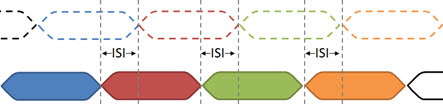# Symbol Duration

As can we easily conclude, a very important determining factor for the ISI is the time duration of the symbol.

If the symbol period (T) is very short compared to the “Delay Spread” (t) the impact is significant (T << t).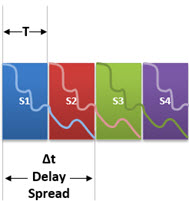But if we can extend the symbols length, most of them will not suffer the impact of ISI (T >> t).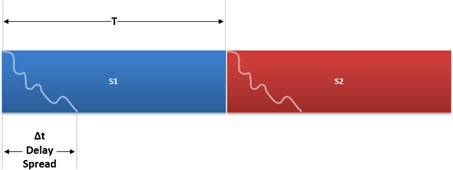One small part of the symbol will continue to be impacted, but for most of its duration, the symbol will remain not affected by reflections propagated in “Multipath”.

That is why the ISI is minimized when we use a higher symbol period (or Lower Symbol Rate).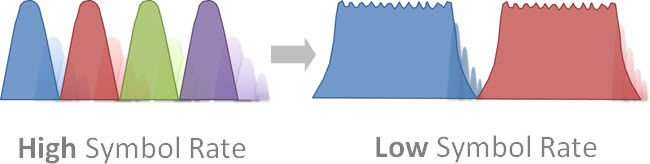# Conclusion

Today we learned, in a very simple way, what is the inter symbol interference present on systems that use symbols for communication.

We saw what causes the interference (the “Delay Spread”, caused by “Multipath”), its consequences, and what we can do to minimize it.

In the next tutorials, We’ll continue to cover topics directly related to LTE (and similar technologies), so that you’ll be able understand all the complex concepts of this technology quite easily.